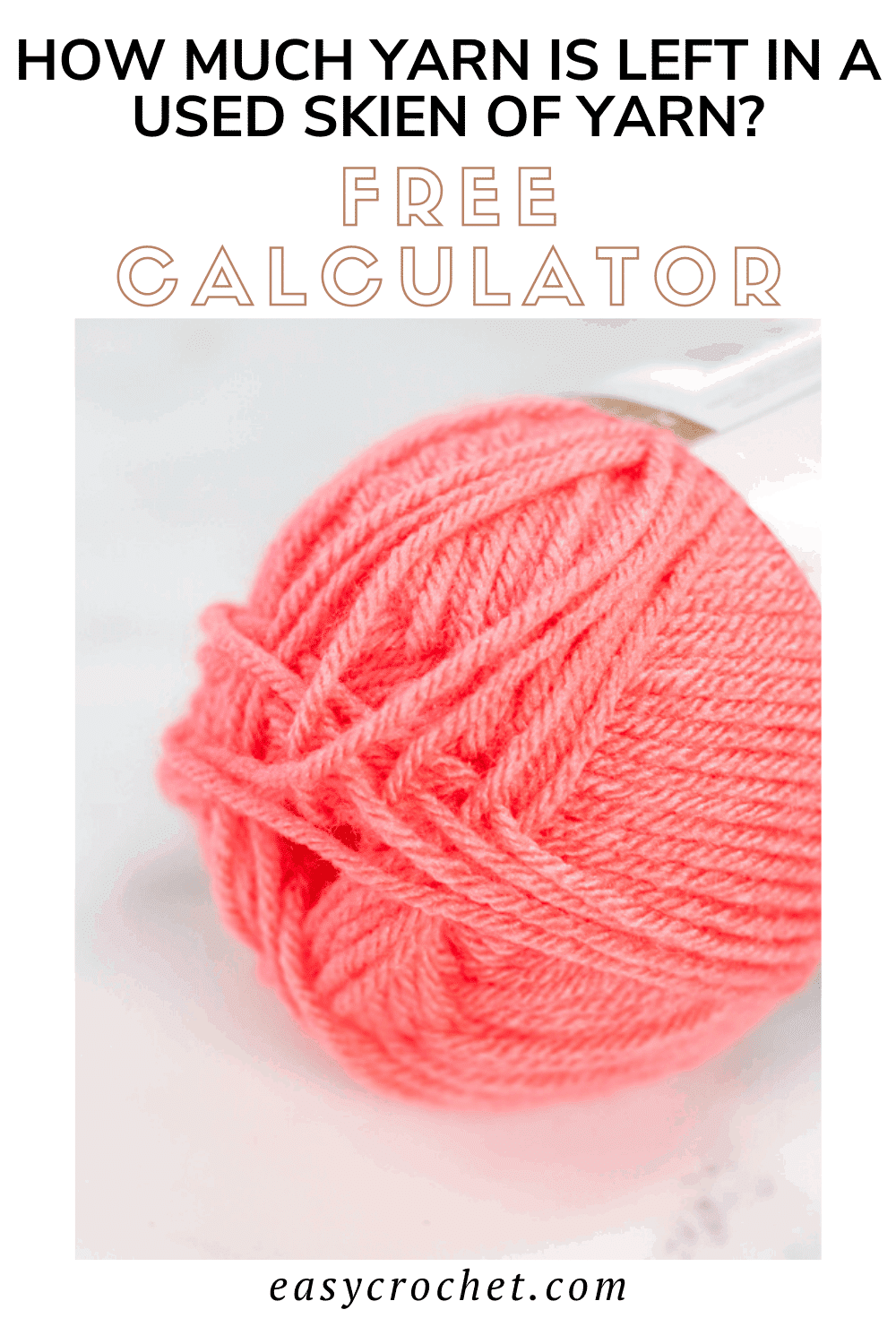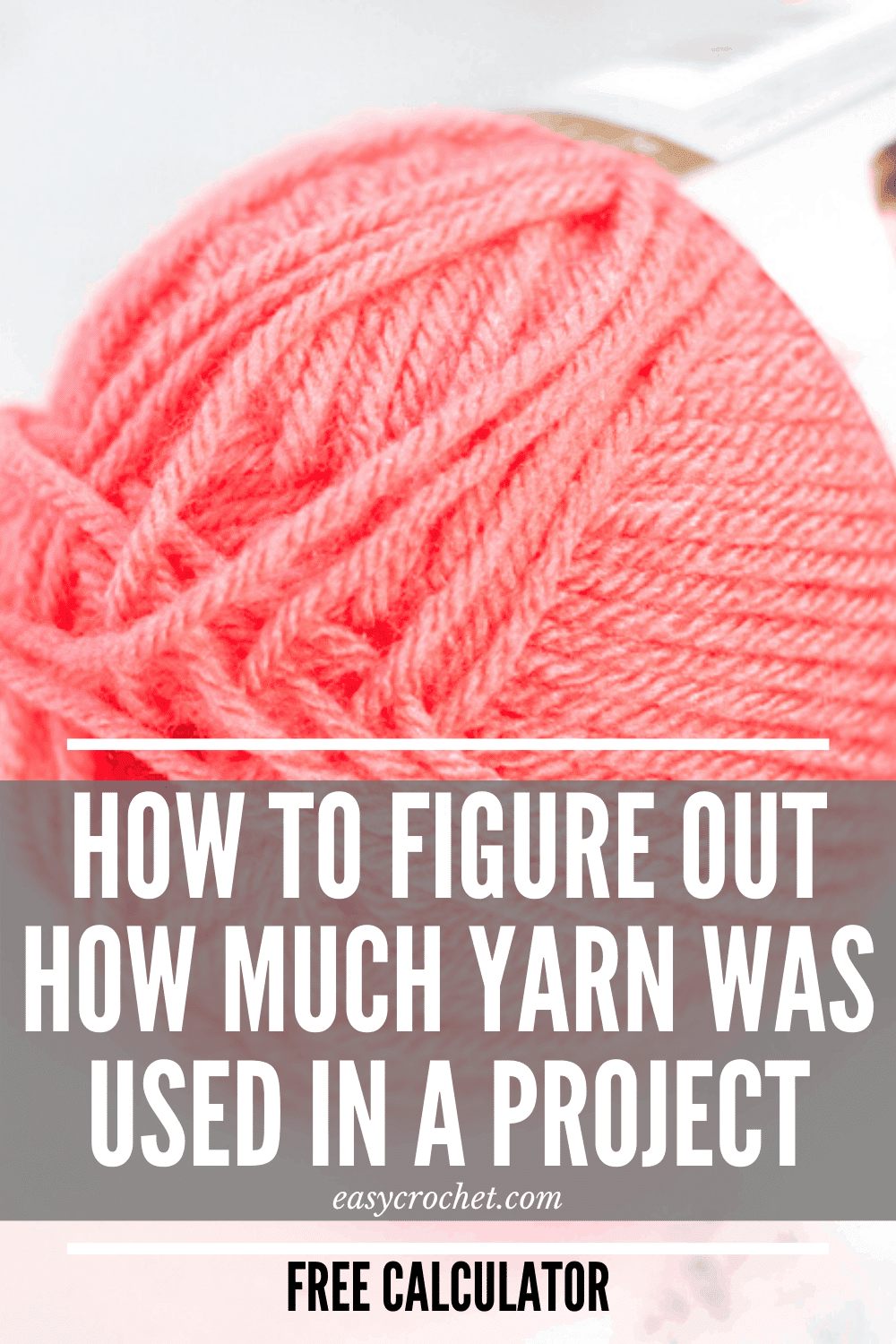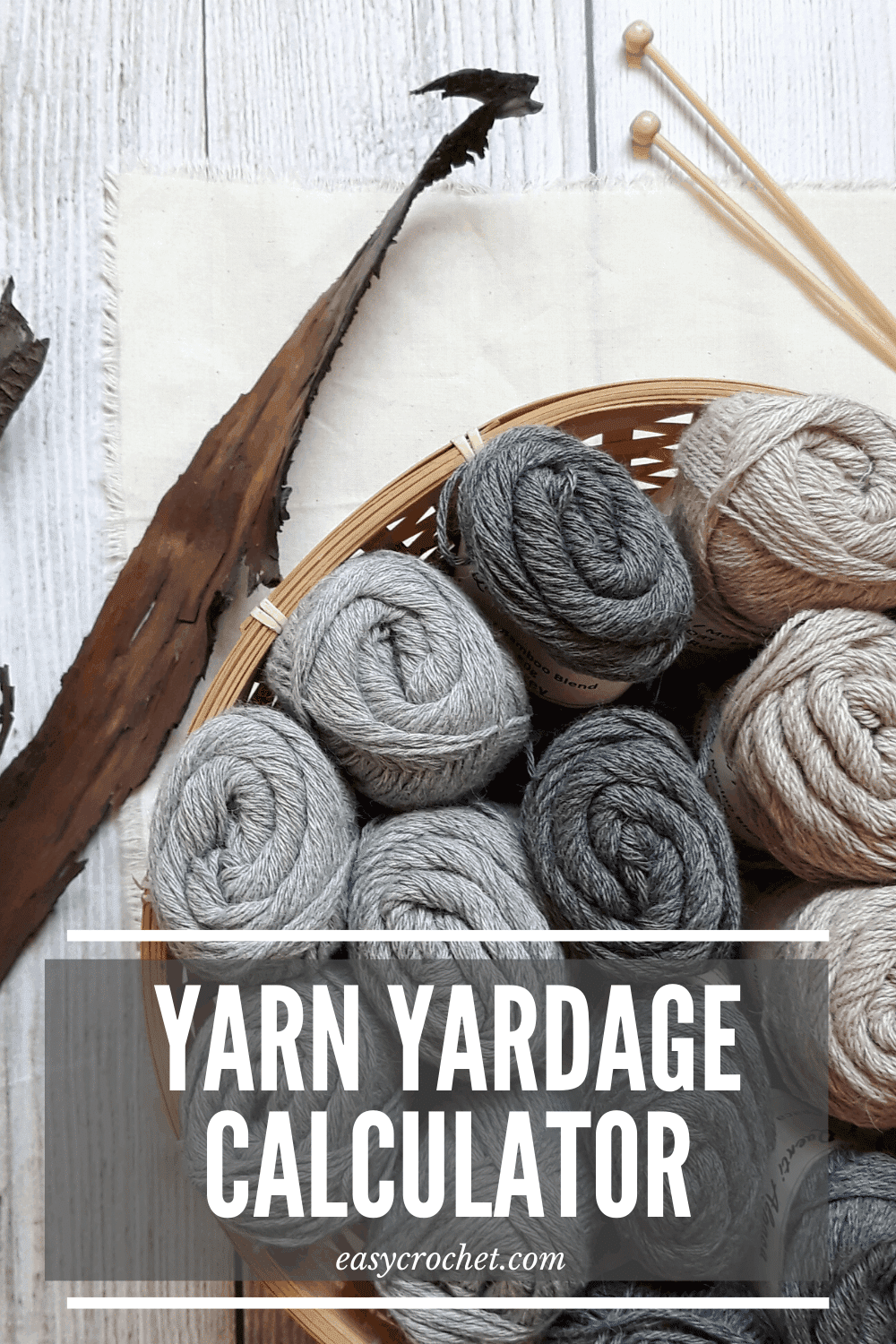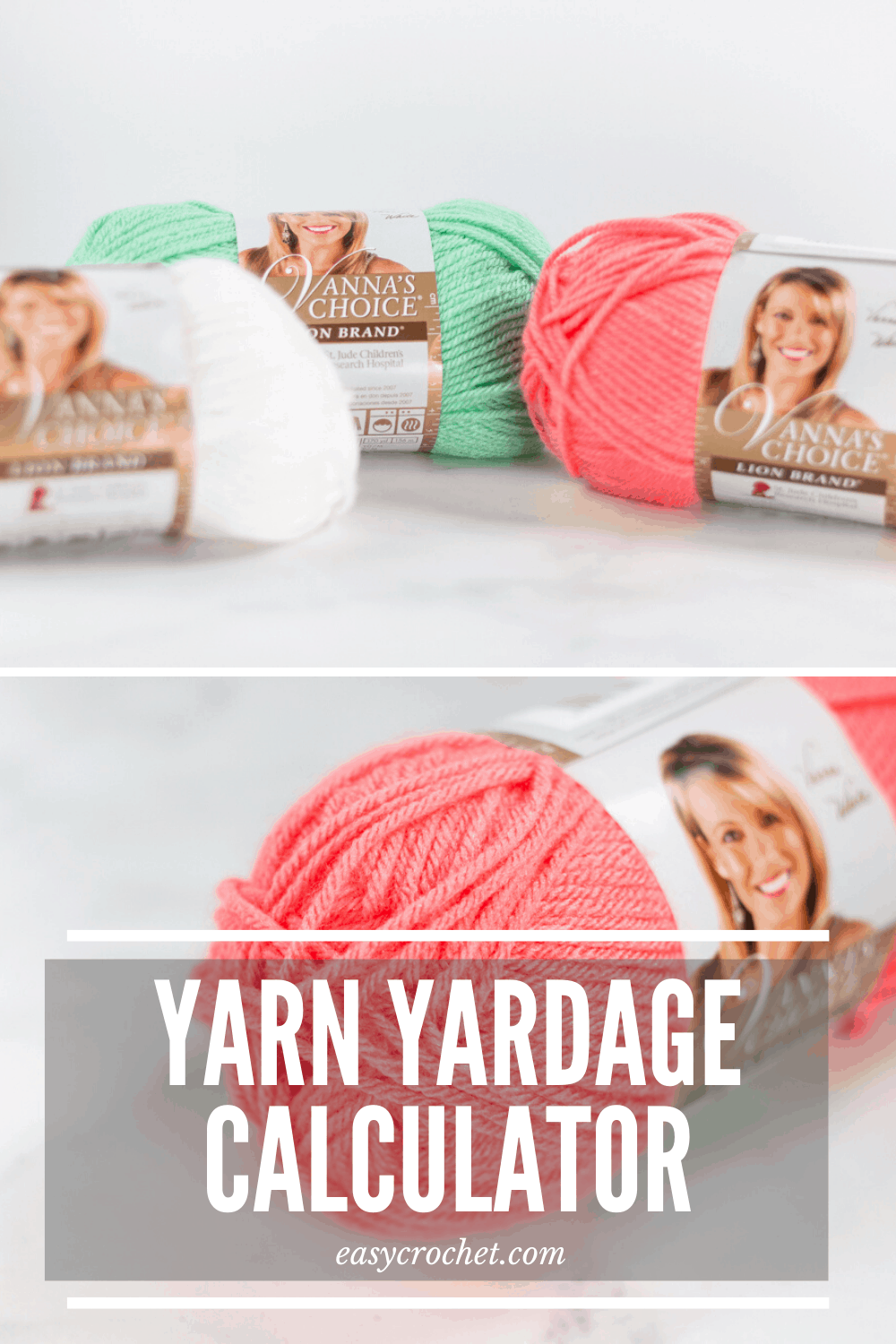# Calculate How Much Yarn is Remaining in a Used SkeinHave you ever wondered how many yards are in a skein of yarn? If so, this yarn calculator shows how much yarn is left inside a partially used skein of yarn.

We all have half-used skeins of yarn lying around. We then wonder how much yarn is left in this skein… well, this simple calculator gives you the answer to that question: “How do I Convert Yarn Weight to Length

Ready to learn how to figure it out?

After finishing a project, we all wonder how many yards are left in that ball of yarn that is left over or you may ask yourself how to calculate yarn yardage from your leftover yarn? The good news is that all you need is a kitchen scale and this tool to calculate yarn yardage by weight.

## How to Calculate Yarn Yardage by Weight

Follow these simple steps & the yarn calculator below to learn how to calculate how much yarn yardage is left in a skein of yarn.

1. First, you need to find the original weight & length of a full skein of the yarn you have. If you don’t have the original sleeve that went around the yarn, you may be able to look up the data on YarnSub.com.
2. If you can’t find the label, then you’re going to need to use this calculator instead.
3. Next, use a kitchen scale to determine the weight of what is left in your yarn ball.
4. Lastly, enter those three values into the calculator below to determine the yardage (or meters) of what you have left.

## Skein Remaining Calculator

### Skein Data

Find the length of yarn remaining in a partially used skein.

(grams or ounces)
(meters or yards)
(grams or ounces)